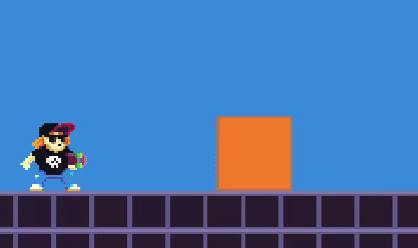Creating enemies and collectable items on Phaser JS - Skate Platformer Game Devlog #08

Written in October 22, 2020 - 🕒 3 min. read

Hey guys, I’m back with another game devlog, it took me a while to make this one because there were a couple of things that I didn’t know how to do (still don’t actually). Watch it below.

Now let’s dive into the code, remember my `dataLayer` loop? This is where we’re going to add our new objects.

``````// in the scene create function
const dataLayer = map.getObjectLayer('data');
dataLayer.objects.forEach((data) => {
const { x, y, name, height, width } = data;

if (name === 'coin') {
// TODO add coin logic here
}

if (['box', 'long_box'].includes(name)) {
// TODO add box logic here
}

if (name === 'enemy2') {
// TODO add enemy logic here
}

});``````

The first new thing is the collectible coins, which is really straightforward, all I needed to do is instantiate a new GameObject and destroy it when colliding with the hero.

``````if (name === 'coin') {
const coin = new Coin({
scene: this,
});

this.physics.add.overlap(this.player, coin, () => {
coin.destroy(true);
});
}``````

Now for the box, the way I wanted it to work was by having the box completely still unless the hero starts pushing it. Just by enabling Arcade Physics on default settings, the hero will exert a force in the box, and the box in the hero, and this is what this looks like:I needed the box to not exert any force into the hero, so to accomplish that I’m using `this.body.setAllowGravity(false)` and `this.body.setImmovable(true)`, as you can see below:

``````constructor({ scene, x, y, asset, frame }) {
super(scene, x, y, asset, frame);

// set physics
this.body.setAllowGravity(false);
this.body.setImmovable(true);
this.body.setAccelerationY(
scene.physics.world.gravity.y
);
}

update(time, delta) {
const gravityY = this.scene.physics.world.gravity.y;
if (this.body.velocity.x !== 0) {
this.body.setAccelerationY(gravityY);
} else if (this.body.acceleration.y !== gravityY) {
this.body.stop();
}
}``````

If you think that hack in the `update` function was the worst part, boy you’re mistaken. Let me present you what I call “the collision shenanigans”.

``````if (['box', 'long_box'].includes(name)) {
let box;
if (name === 'box') {
box = new Box({
scene: this,
x,
y,
});
} else {
box = new LongBox({
scene: this,
x,
y,
});
}

// Add colliders that forces the box to stop
this.physics.add.collider(dynamicLayers.ground, box, () => {
box.body.stop();
});
this.physics.add.collider(dynamicLayers.elements, box, () => {
box.body.stop();
});
this.physics.add.collider(mapGroundColliders, box, () => {
box.body.stop();
});
this.physics.add.collider(mapElementsColliders, box, () => {
box.body.stop();
});

// Handles pushing the box
this.physics.add.collider(box, this.player, () => {
if (
this.player.body.touching.right
&& box.body.touching.left
) {
this.player.body.velocity.x = 30;
box.body.setVelocityX(
this.player.body.velocity.x
);
}

if (
this.player.body.touching.left
&& box.body.touching.right
) {
this.player.body.velocity.x = -30;
box.body.setVelocityX(
this.player.body.velocity.x
);
}
});
}``````

And last but not least, the enemy, which is pretty much the same as the `coin`, but instead of destroying the enemy on collision, I made the hero blink for a couple of seconds.

``````if (name === 'enemy2') {
const enemy2 = new Enemy2({
scene: this,
});

this.player,
enemy2,
(objectA, objectB) => {
targets: player,
alpha: 0,
ease: 'Cubic.easeOut',
duration: 120,
repeat: 5,
yoyo: true,
});
}
);
}``````

That’s all for today! Stay tuned for next week when I’m going to show you how to build the enemy movement AI. Don’t forget to like my video and subscribe to my channel 😊.

Tags: